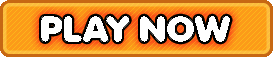Bookmark

# Number Ninja Basic Facts, Integers, TwoStep Equations

4.6 stars (3,870 plays / 17 votes)Number Ninja | Basic Facts, Integers, & Two-Step EquationsOpen boxes to find numbers that fit into the math problem.
4.6 stars out of 5 (3,870 plays / 17 votes)

KidzSearch > Games > Math GamesTags:  math    negative    missing    number    addition    subtraction    addend    elementary    math    math    fun    math    integer    equation    two    step    equation    numbersMember of the American Library Association  Copyright 2005-2020 KidzSearch.com